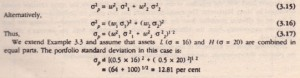Select Page

Zero Correlation

(p = 0) When the returns on two assets. are uncorrelated, their correlation and hence, co-variance terms become zero. In this case, portfolio variance is the sum of the square of standard deviation of each asset weighted by its proportion in the Portfolio. Thus,Again, diversification has helped in reducing the risk. The risk of the portfolio is less dun the risk or either or me two assets in the portfolio.
In brief, the risk of an asset in the portfolio is the contribution it makes to overall risk. The true risk of an asset may be judged at the level of a portfolio only. A highly risky asset may act as portfolio risk stabilizer when returns on it bear low or negative correlation with returns on other assets in the portfolio.